# Filter Bank: What is it? (DCT, Polyphase, Gabor, Mel And FBMC)

Contents

## What is a Filter Bank?

In image or signal processing, a Filter Bank is defined as an arrangement of bandpass filters that split the input signal into a set of analysis signals, each one carrying a single frequency sub-band of the original signal.

In other words, a class of systems that generates scaling and wavelet function are known as filter banks. The below figure shows the simple structure of a filter bank. It is a set of bandpass filters with each filter centered at a different frequency.

A digital filter bank is a collection of filters having a common input or output. In digital signal processing, the term filter bank is often applied to the bank of receivers.

A filter bank is used to performed various tasks like bandwidth reduction, combinations of the common operations of spectral translation, spectral composition and decompositions of signals, and sample rate changes, etc. since most filter banks include various sampling rates, they are also referred to as multi-rate systems.

Filter banks play an important role in modern signal and image processing applications such as audio and image coding.

## How does a Filter Bank work?

A filter bank separates or splits the input signal into multiple components. The process of separating the input signals into multiple components is known as analysis. The output of the analysis is referred to as a sub-band of the original signal.

Now, the Filter bank attenuates the components of the signal differently and reconstructed them into an improved version of the original signal.  This reconstruction process is known as synthesis.

For example, if we have an input audio signal x(n), then filter banks separate this input audio signal into a set of analysis signals i.e., x1(n), x2(n), x3(n), etc…, each of these set of analysis signals corresponds to a different region in the spectrum of the input signal x(n).

This set of analysis signals x1(n), x2(n), x3(n)… can be obtained by filter banks with bandwidths BW1, BW2, BW3… and centre frequencies fc1, fc2, fc3… respectively.

The below figure shows the frequency response of a Filter Bank in which bands of a three-band filter bank do not overlap, but are lined up one after the other with adjacent band edges touching each other. These three bands span the frequency range from fcl1 = 0 Hz to fch3 = fmax.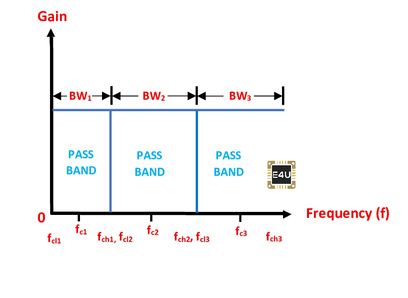Frequency Response of a Filter Bank and Bandpass Filter

### Analysis and Synthesis Filter Bank

There are two main types of filter banks. An analysis filter bank and a synthesis filter bank. An analysis filter bank is a set of analysis filters Hk(n) which splits an input signal into M sub-band signals Xk(n) and a synthesis filter bank is a set of M synthesis filters Fk(z) which combine M signal Yk(n) into a reconstructed signal x^(n) as shown in the below figure.

The analysis filter bank decomposes the input signal to a different sub-band with a different frequency spectrum and the synthesis filter bank reconstructs the different sub-band signal and generates a modified version of the original signal.

## Types of Filter Banks

There are several types of filter banks available. let’s discuss one by one.

## DCT Filter Banks

DCT (Discrete Cosine Transform) Filter Banks is linear transform filter banks used to compress data in sets of discrete DCT blocks. DCT Filter Banks are similar to the DFT filter banks but it uses only real numbers.

The representation of the DCT decomposition as a filter bank or a bank of filters is shown in the below figure. This describes the simplification of the DCT as a filter bank and modifications of sub-bands for image modifications.

As shown, we apply DCT to blocks of 8*8 pixels.  we have one filter for each sub-band of the DCT. Here, 8 samples coming out from the filter are given to the down sampler. we have a block-wise representation that corresponds to down sampling by a factor of 8 for each sub-band.

This down sampler only passes every 8th sample and blocks other samples hence, we get only one sample.

So, DCT works as a bank of filters as we get smaller sub-band images in sets of discrete DCT samples.

### Advantages of a DCT Filter Bank

Some of the advantages of a DCT as filter banks include:

• We can represent a DCT Filter Bank as a square matrix multiplication since the square matrix is invertible hence, the DCT filter bank is invertible. So, the DCT filter bank can be used to obtain a synthesis filter bank for perfect reconstruction.
• The DCT is more efficient computationally.

## Polyphase Filter Banks

A polyphase filter bank is a multi-rate filter structure combined with a DFT to extracts sub-bands from an input signal. It is simply a computational structure for applying resampling and filtering to a signal.

In image or signal processing, an instrument needs to do Discrete Fourier Transform (DFT) on input signals. This Discrete Fourier Transform (DFT) on an input signal has two drawbacks one is leakage and the second is scalloping loss.

The Polyphase Filter Banks is a technique used to overcome the drawbacks of DFT. The Polyphase Filter Banks are used to creates a flat response across the channel, also provide excellent suppression of out-of-band signals.

A polyphase filter bank implements the DFT to modulate a prototype filter and computes summation and filtered time-domain data from the number of DFT stages.

Mathematically, the weighting(window) function can be written as,Where h(n+pN) is the sub-filter coefficients that correspond to P-tap polyphase sub-filters. The N such polyphase sub-filters together with the number of DFT stage are collectively called a “Polyphase Filter Bank (PFB)”. The structure of a polyphase filter bank with P=3 taps and N sub-filters and the frequency response of a Polyphase Filter Bank is shown in the below image.

## Gabor Filter Banks

In image or signal processing, Gabor Filter banks are defined as an array of functions for generating and filtering images using a bank of Gabor filters in a frequency domain.

Gabor Filter banks is a linear filter bank that is used for texture analysis, which usually means that it analyses whether there is any specific frequency band in the image in particular directions in a localized region around the region of analysis.

Gabor filter is a convolution filter representing a combination of sinusoidal and gaussian components. The sinusoidal component provides directions and the gaussian component provides weights. The Gabor filter has a real and imaginary component that represents orthogonal directions.

Mathematically, it can be expressed as,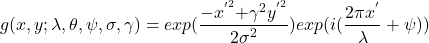So, Gabor is a convolution filter where we can change wavelength, orientation, phase offset, aspect ratio, etc. By changing these we can extract any number of Gabor filters.

By using real parts of a variety of different Gabor filter kernels we can filter the images. These Gabor filter kernels contain functions to extract amplitudes, phases, real and imaginary parts. The image response for different Gabor filter kernels at different frequencies and a frequency response of a Gabor filter bank is shown in the below images.

Gabor Filter Banks can compensate DC component also feature extraction and convolution can be done by using only one function. Frequency and orientation representations of the Gabor filter banks are similar to the human visual system.

## Mel Filter Banks

Mel Filter Banks is a triangular filter bank that works similar to the human ears perception of sound which is more discriminative at lower frequencies and less discriminative at higher frequencies.

Mel Filter Banks are used to provide a better resolution at low frequencies and less resolution at high frequencies. It captures the energy at each critical frequency band and gives approximates the spectrum shape.  The below block diagram shows how Mel Filter Banks works to obtain Mel-Frequency Cepstral Coefficients.

As shown, a speech signal is applied to a pre-emphasis filter, this filter sectionalized speech signal into (overlapping) frames then a window function is applied to each frame. Note that a Pre-emphasis filter is useful for 1. to balance the frequency spectrum as high frequencies usually have smaller magnitudes compared to lower frequencies, 2. to improve Signal-to-Noise Ratio (SNR).

Now, we need to separate the speech signal into short-time frames. To do that a Discrete Fourier Transform (DFT) on each frame (especially a Short-Time Fourier Transform) is applied and calculate the energy or power spectrum. Hence, we get an approximation of the frequency spectrum of the speech signal by cascading adjacent frames.

Now, to obtain Mel-Frequency Cepstral Coefficients (MFCC), an Inverse Discrete Fourier Transform (IDFT) or a Discrete Cosine Transform (DCT) is applied to the Mel Filter Banks, thus we get several MFCCs coefficients while the rest are discarded.

Then, the final step to computing Mel filter Banks is applying triangular filters on a Mel-scale to extract frequency bands. We can convert Mel(m) and frequency(f)(Hertz) by using the following equations.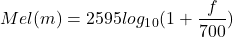Each filter in the Mel Filter Bank is a triangular filter having a frequency response of 1 at the centre frequency and decreases linearly towards 0 till it reaches the centre frequencies of the two adjacent filters.

Mathematically, the Mel spectrum can be expressed as,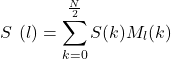For each Mel spectrum, we get a triangular frequency response. Hence, the set of Mel spectrum i.e., m1, m2, m3,……,mM we get a whole range of triangular frequency responses. The frequency response of a Mel Filter bank is shown in the below image.

## Filter Bank Multicarrier (FBMC)

FBMC stands for Filter Bank Multicarrier is defined as a form of multi-carrier modulation that transmits the data by splitting it into several components and transmit each of these components through separate carries signals. FBMC divides the frequency spectrum into narrow subchannels.

One of the limitations of OFDM is that the spectral localization of the subcarriers is weak. This results in spectral leakage and hence it interferes with unsynchronized signals.

FBMC is an alternative transmission method that is used to overcome the limitations of OFDM by using high-quality filters that reject both ingress and egress noises.

The comparison of frequency response between OFDM Vs FBMC is shown in the below graph. As shown, when carriers are modulated in an OFDM system, side-lobes are spread out either side but with the FBMC system these side-lobes are removed and we get cleaner carriers.

In FBMC systems, FIR filters are preferred over IIR filters, one reason behind that FIR filters have a linear phase whereas IIR does not. FBMC can provide higher data rates within a given radio spectrum band.

## DFT Filter Banks

DFT Filter Banks is Fourier transform filter bank which can be constructed using length M FIR bandpass filters where each bandpass filter is implemented as a demodulator followed by a running-sum lowpass filter.

DFT Filter Banks are similar to the DCT filter banks but it uses complex numbers whereas DCT filter banks use only real numbers.

The below system diagram shows the N-channel DFT filter bank constructed using length M FIR running-sum lowpass filters.

The kth channel computes from the above system diagram are given by,

(1)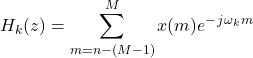As we know that the length M Discrete Fourier Transform is defined as,

(2)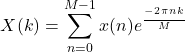Where, X(k) is in the frequency domain

x(n) is in the time domain

Now, compare equation (1) and (2), we can see that the output of the filter bank i.e.,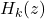(where, k=0,1, 2…, M-1) is the DFT of the input signal when n=M-1, it is given by

(3)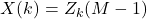In other words, the output of the filter bank at time n=M-1 is equal to the DFT of the first M samples of x(n) (where, n=0,1, 2…, M-1). Hence, we can say that the output of the all-filter bank channels at time M-1 is the DFT of the input signal from time 0 through M-1.

Hence, the DFT system is like a filter bank with analysis filters. The frequency response of a DFT filter bank is shown in the below figure.

### Uniform DFT Filter Bank

An analysis filter bank with M (M>1) filters is said to be a uniform DFT filter bank if all the filters are obtained from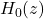according to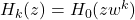, where, 0<=k<=M-1. Here,is called a prototype filter.

Note that,which means that the frequency response ofis a uniformly shifted version of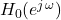. The below figure the typical frequency response of a uniform DFT filter bank whereis taken to be a low-pass filter.

## Advantages of Filter Bank

Some of the advantages of a filter bank include:

• High resolution with low spectral leakage
• More stable peaks
• The more accurate and consistent noise floor
• By using filter banks, we can set channelizers to get different resolutions at different frequency bands. This is used in audio spectral analysis.

## Applications of Filter Banks

Some of the applications of the filter banks include:

• The filter bank is used to compress the signal when some particular frequencies are more important than other frequencies. They are used in speech processing, image compression, communications systems, antenna systems, analog voice privacy systems, and in the digital audio industry.
• The filter bank can be used as a graphic equalizer, which can attenuate the components of the signal differently and reconstructed them into an improved version of the original signal.
• Filter banks are used for trans multiplexers, sub-band adaptive filtering, vocoder, etc. Here, the vocoder uses a filter bank to determine and control the amplitude of the sub-bands of the carrier signal.
• DCT filter Banks are used in JPEG image compression and modifications of sub-bands for image modifications.
• DCT Filter banks are used in audio signal processing, digital audio, digital radio, speech processing, etc.
• FBMC (Filter Bank Multicarrier) can be used as a tool for spectrum sensing.
• In document image processing, Gabor filter banks are used for identifying the script of a word in a multilingual document.
• Gabor filter banks are widely used for decomposing an image into oriented sub bands such as texture analysis, edge and texture detection, feature extraction, object and shape recognition, scene segmentation, etc.

Want To Learn Faster? 🎓
Get electrical articles delivered to your inbox every week.
No credit card required—it’s 100% free.